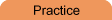Home    |    Teacher    |    Parents    |    Glossary    |    About UsEmail this page to a friendSAT Quantitative Comparisons
Information provided by www.kaptest.comThere are 15 Quantitative Comparison, or QC, questions on the quantitative section of the SAT, so QCs account for 25 percent of your quantitative score. The math tested on QCs is the same as that tested on Regular Math questions: junior high school level arithmetic, algebra and geometry.

QCs don't ask you to solve for a particular value. Instead they ask you to compare two quantities and determine which, if either, is larger. Because of their unusual format, QCs can seem daunting at first, but once you become familiar with this question type, you'll actually find them easier and faster to do than regular Problem Solving questions. Each QC will show you two quantities or mathematical expressions—one in Column A and one in Column B. Sometimes additional information will be provided about one or both quantities. This information will be centered between the two columns, and is essential to answering the question.

The basic directions and answer choice options for QCs will remain the same. So take a moment to familiarize yourself with them now.

 Directions: The following question consists of two quantities, one in Column A and one in Column B. You are to compare the two quantities and decide whether the quantity in Column A is greater, the quantity in Column B is greater, the two quantities are equal, or the relationship cannot be determined from the information given.

(Note: the first three answer choices represent definite relationships between the quantities in Columns A and B, but the last answer choice indicates that a relationship cannot be determined. So the last answer choice (choice D) can never be correct if only numbers appear for the two quantities, since it indicates that more than one relationship can exist between the two quantities. The relationship between any two numbers is unchanging.)

### Sample Question #1

x
and y are positive integers.
3x + 6y = 180,252

Column A                                          Column B

The maximum possible                 The maximum possible
value of x                                        value of y

The quantity in Column A is greater.
The quantity in Column B is greater.
The two quantities are equal.
The relationship cannot be determined from the information given.